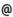## "Babes-Bolyai" University of Cluj-Napoca Faculty of Mathematics and Computer Science

 Ordinary differential equations and dynamical systems (2)
 Code Semes-ter Hours: C+S+L Credits Type Section ME002 6 2+1+0 4 optional Matematică-Informatică ME002 6 2+1+0 6 compulsory Matematici aplicate
 Teaching Staff in Charge
 Prof. PETRUSEL Adrian Olimpiu, Ph.D., petruselmath.ubbcluj.ro
 Assoc.Prof. BEGE Antal, Ph.D., begemath.ubbcluj.ro
 Aims 1. The study of the Fredholm and Volterra types integral equations. 2. The study of the boundary value problems for second order differential equations. 3. The study of some qualitative properties of the solutions of linear differential equations of second order. 4. The study of basic notions and results from the thory of Dynamical Systems. 4. The study of the stability behavior of differential systems.
 Content 1. Volterra integral equations. Existence and uniqueness theorems. 2. Fredholm integral equations. Existence and uniqueness theorems. 3. Boundary value problems for differential equations of second order. 4. Maximum and minimum principles for linear differential equations of second order. 5. Zero-points for the solutions of second order linear differential equations. 6. Dynamical systems. Basic notions and results. 7. Stability theory for systems of differential equations. 8. First order partial differential equations.
 References 1. I.A.RUS, Ecuatii diferentiale, ecuatii integrale si sisteme dinamice (Transilvania Press, Cluj-Napoca, 1996). 2. I.A.RUS, P.PAVEL, Ecuatii diferentiale, Ed.Didactica, Bucuresti, 1982. 3. V.BARBU, Ecuatii diferentiale, Ed. Junimea, Iasi, 1985. 4. D.V.IONESCU, Ecuatii diferentiale si integrale, Ed.Didactica, Bucuresti, 1972 5. S.D. CHATERJI, Cours d'Analyse 3- Equations differentielles ordinaires et aux derivees partielles, Press polytech. et univ. de Lausanne, 1998. 6. L. PERKO, Differential equations and dynamical systems, Springer, Berlin, 1991.
 Assessment Seminar and lab activities during the semester: two written tests (80%) and a lab test (20%).